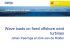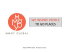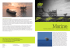# Physical simulation of resonant wave run

## Transcription

Physical simulation of resonant wave run
```Nonlin. Processes Geophys., 20, 35–40, 2013
www.nonlin-processes-geophys.net/20/35/2013/
doi:10.5194/npg-20-35-2013
Nonlinear Processes
in Geophysics
Physical simulation of resonant wave run-up on a beach
A. Ezersky1 , N. Abcha1 , and E. Pelinovsky2,3,4
1 CNRS,
UMR6143 – Morphodynamique Continentale et Côtière (M2C), Université Caen Basse, Normandie,
24 rue des Tilleuls, 14000 Caen, France
2 Institute of Applied Physics, 46, Ul’janov St., Nizhny Novgorod 603950, Russia
3 Nizhny Novgorod State Technical University, Nizhny Novgorod, Russia
4 National Research University – Higher School of Economics, Nizhny Novgorod, Russia
Correspondence to: E. Pelinovsky ([email protected])
Received: 27 July 2012 – Revised: 23 November 2012 – Accepted: 26 November 2012 – Published: 9 January 2013
Abstract. Nonlinear wave run-up on the beach caused by
a harmonic wave maker located at some distance from the
shore line is studied experimentally. It is revealed that under
certain wave excitation frequencies, a significant increase in
run-up amplification is observed. It is found that this amplification is due to the excitation of resonant mode in the region between the shoreline and wave maker. Frequency and
magnitude of the maximum amplification are in good correlation with the numerical calculation results represented in
the paper (Stefanakis et al., 2011). These effects are very important for understanding the nature of rogue waves in the
coastal zone.
1
Introduction
Recent huge tsunamis demonstrate nonlinear behaviour on
the coast leading to strong impact. It was also revealed recently that the number of abnormally large and suddenly appearing waves (rogue waves) observed in the coastal zone is
sufficiently larger than Gaussian statistics predicts (Nikolkina and Didenkulova, 2011, 2012). Analysis of tsunami
records showed that reflections due to bottom topography
may result in the appearance of resonant mode in the coastal
zone, see for instance Neetu et al. (2011). The study of the
coastal rogue waves is based on the nonlinear theory of shallow water (Kharif et al., 2009; Didenkulova and Pelinovsky,
2011; Slunyaev et al., 2011). To characterize the impact of
waves on coastal infrastructure, the systematic study of runup process is undertaken, and a lot of papers summarizing the progress in the analytical solutions of the nonlinear shallow-water theory have been published by now (see
for instance Carrier and Greenspan, 1958; Pelinovsky, 1982;
Synolakis, 1987; Pelinovsky and Mazova, 1992; Carrier et
al., 2003; Kânoğlu and Synolakis, 2006; Didenkulova et al.,
2007; Madsen and Fuhrman, 2008; Kajiura, 1977). Recently,
Stefanakis et al. (2011), on the basis of numerical simulations
of the nonlinear shallow-water equations, pointed out the existence of resonance effects in the process of the long wave
run-up. It should be noted that such resonance effects were
predicted in Antuono and Brocchini (2010) in the framework
of linear theory.
The main result of Stefanakis et al. (2011) is that at a certain frequency of the waves there exists a significant increase
in the run-up amplitude. According to calculations, the maximal run-up height can be 50 times greater than the free surface oscillation amplitude used as the boundary condition in
the numerical calculations. It should be emphasized that the
estimations presented in the different papers cited above provide us an order of maximal run-up height much less than
in Stefanakis et al. (2011). It was established in Stefanakis
et al. (2011) that the wave period for which maximal run-up
amplification is observed depends on the slope of the bottom
and the depth of water in the place where the waves are excited. This period is much larger than the “natural period” –
time needed for perturbations to run from the point of excitation to the shoreline and return back. Results obtained in
Stefanakis et al. (2011) pose a lot of questions. That is why
in order to investigate the physical mechanisms of run-up
amplification, needed to explain the coastal extreme rouge
waves, we carried out a physical simulation of this process
in the hydrodynamic channel with an inclined bottom. The
simulation is carried out in such a way that its results can be
Published by Copernicus Publications on behalf of the European Geosciences Union & the American Geophysical Union.
36
A. Ezersky et al.: Physical simulation of resonant wave run-up on a beach
used for comparison with numerical calculations presented
in Stefanakis et al. (2011).
Before the presentation of experimental results, we give
briefly some theoretical estimates. The long wave run-up on
a plane beach is described by the nonlinear shallow-water
equations:
∂u
∂η
∂u
+u
+g
= 0 and
∂t
∂x
∂x
∂
∂η
, +
(1)
(u (h + η)) = 0
∂t
∂x
where u is the depth-averaged velocity, h = h(x) is the unperturbed water depth, η = η(x, t) is the free surface displacement, and g is the acceleration of gravity. For linear
variation of water depth, h = xθ (θ is the tangent of bottom
slope angle, x is horizontal coordinate), solutions of these
equations may be found using the hodograph transformation
(details can be found in papers Carrier and Greenspan, 1958;
Pelinovsky, 1982; Synolakis, 1987; Pelinovsky and Mazova,
1992; Carrier et al., 2003; Kânoğlu and Synolakis, 2006; Didenkulova et al., 2007; Madsen and Fuhrman, 2008; Kajiura,
1977). For instance, if the incident wave far from the shore
is a monochromatic wave of frequency ω, the solution is presented in the following form:
1 ∂ϕ
1 ∂ϕ
2
−u , u =
,
η=
2g ∂λ
σ ∂σ
!
∂ϕ
λ−u
σ2
1
, t=
− u2 −
, and
x=
2gθ ∂λ
2
gθ
2gθ 2
RJ0 (ωλ/θg) sin (ωλ/θg)
(2)
ω
where ϕ(σ, λ) is an auxiliary function, σ = 2[g(h +
η)]1/2 , andJ0 is the Bessel function of zero order. Generally
speaking, wave field in the vicinity of the shoreline (x = 0) is
not monochromatic, but if the nonlinear effects are ignored,
the solution (Eq. 2) is written in the following form:
q
2
η (x, t) = RJ0
4ω x/gθ cos (ωt) .
(3)
,ϕ (σ, λ) =
It is a standing wave with knots coinciding with zeros of the
Bessel function. The constant R plays the role of the amplitude of the water oscillation on “unmoved” shore (x = 0). As
it can be rigorously shown from Eq. (2), R is the maximal
run-up amplitude of the wave on the coast (moving shoreline) (Pelinovsky and Mazova, 1992). Far from the shoreline
the wave is always linear because its amplitude is much less
than water depth. Using asymptotics of the Bessel functions
for large values of arguments, we can select the incident wave
with amplitude A at the distance L from the shoreline and obtain the amplification ratio in the following form (Pelinovsky,
1982; Pelinovsky and Mazova, 1992; Madsen and Fuhrman,
2008):
1/4
p
√ (4)
= 2π 2L/λ0 ,
R/A = 2 π h0 ω2 /gθ 2
Nonlin. Processes Geophys., 20, 35–40, 2013
where λ0 is the wavelength on the isobath h0 located on distance L from the shore. It is important to point out that the
asymptotic (Eq. 4) is valid for large values of L/λ0 and represents nonlinear amplification ration obtained directly from
Eq. (2). In the case of small values of L/λ0 , correct selection
of incident and reflected waves is possible if the beach of
length L is matched with flat bottom. In this case the amplification ratio is given by Pelinovsky (1982), Pelinovsky and
Mazova (1992), Madsen and Fuhrman (2008), and Keller and
Keller (1964):
q
(5)
R/A = 2/ J02 (4π L/λ0 ) + J12 (4π L/λ0 ).
Comparison of Eqs. (4) and (5) is given in Fig. 1. As it can be
seen, the resonance effects are very small because the “resonator” (0 < x < L) is open from one boundary. For tsunami
application, the characteristics of the incident wave very often are unknown. Meanwhile, a lot of buoys are now installed
along the coasts (tide-gauge stations and DART buoys), and
tsunami records on such buoys can be considered as input for
solving the run-up problem. In the simplified geometry of a
plane beach, the buoy measures the standing wave (Eqs. 2
or 3), and in the case of small wave amplitude, free surface
oscillations are described by:
q
η (L, t) = a cos (ωt) , and a = RJ0
4ω2 L/gθ .
(6)
The amplification factor computed for the tsunami wave
propagated from the buoy to the coast,
q
−1
2
C = R/a = J0
4ω L/gθ ,
(7)
has evident resonant properties and can be very large. Such
big values of local amplification factors have been discovered in a recent paper (Stefanakis et al., 2011) where the result was obtained by numerical computation. In fact, it is not
the resonance caused by a particular frequency of incoming
wave but instead a ratio between the run-up and the wave amplitude measured at a particular distance from the coastline.
This amplification of run-up ratio can be very important for
prediction of tsunami behaviour based on data of the nearest
buoy. Following Stefanakis et al. (2011), we call amplification of run-up ratio at particular frequencies as resonance.
Analytical and numerical results described above are examined below in physical experiment.
Experiments were performed in a long hydrodynamic
flume of 0.5 m width. The flume is equipped with a wave
maker controlled by computer. To simulate an inclined bottom, a PVC plate with thickness of 1 cm is used. The
plate is placed at different angles relative to the horizontal bottom of the flume in the vicinity of the wave maker
(see Fig. 2). Three series of experiments have been performed for water depth h near the wave maker and length
L, (h0 =0.245 m, L =145.8 m, θ = 0.168) (h0 = 0, 26 m,
www.nonlin-processes-geophys.net/20/35/2013/
A. Ezersky et al.: Physical simulation of resonant wave run-up on a beach
37
Fig. 2. Schema of experiment: resistive probes (P1, P2), high-speed
video camera (2), wave maker (3), inclined bottom (4).
Fig. 1. Amplification run-up ratio: dashed line corresponds to the
constant beach slope Eq. (4); solid line obtained for inclined beach
matched with horizontal bottom Eq. (5).
L = 135 m, θ = 0.192) and (h0 = 0.32 m, L = 121.5 m, θ =
0.263). Two resistive probes (P1, P2, see Fig. 2) are used to
measure a displacement of water surface. The first of them,
probe P1, is placed at a fixed distance of 1 cm from the wave
maker. The position of a mobile one, P2, is changed using
a coordinate system. Probes allow us to investigate the amplitude of free surface oscillations and phase of oscillation
(probe P1 is used as a “clock”) along the flume. Run-up
height is determined by processing a movie that is shot by
a high-speed camera mounted as shown in Fig. 2. The wave
maker allows us to excite a harmonic wave of a given frequency and it works in two regimes. The first regime allows
controlling the amplitude of wave maker displacement, the
second one allows controlling the amplitude of force applied
to the wave maker. In both regimes it is not possible to control free surface displacement, as was done in the numerical
experiment. That is why to study the run-up amplification,
simultaneous measurements of the amplitude of free surface
displacement near the wave maker and maximal run-up are
carried out for different frequencies of excitation.
The force control regime was chosen for all experiments.
The frequency dependence of the amplitude of free surface
displacement near the wave maker (a), maximal run-up (R)
and coefficient of run-up amplification are shown in Fig. 3
for the slope of the bottom θ = tan(α) = 0.263. The amplitude of force applied to the wave maker was the same for all
frequencies. The amplitude of free surface displacement has
peaks at frequencies f1 = 0.44 Hz and f2 = 0.78 Hz. They
are the resonant frequencies of the system. The maximal runup does not have sharp peaks, only a small increase of R in
the vicinity of f1 and f2 is observed (Fig. 3b). The coefficient of run-up amplification (Fig. 3c) increases very sharply
in the vicinity of f3 = 0.28 Hz and f4 = 0.64 Hz. It is evident that maximal amplification of run-up is observed for
frequencies corresponding to the minimal amplitude a. In
www.nonlin-processes-geophys.net/20/35/2013/
the vicinity of the wave maker, the free surface amplitude
is sufficiently small and the signal is very noisy. That is why
the coefficient of run-up amplification requires rather delicate measurements of free surface displacement: a band-pass
filter was used to measure the amplitude of the harmonic corresponding to wave maker forcing.
It is important to note that the frequency and the coefficient
of maximal amplification do not depend on the method of
wave excitation. Results presented in Fig. 3 were obtained for
the force-controlled regime of the wave maker; the same results for coefficient of run-up amplification were obtained for
the displacement controlled regime. It should be noted that if
the amplitude of force applied to the wave maker increases,
the coefficient of run-up amplification has small changes up
to the appearance of wave breaking near the coastline.
Using movie recorded by a high-speed camera, we estimate the so-called wave-breaking number “Br” (Denissenko
2
et al., 2011): Br = VgR , where V is velocity of fluid particles
at x = 0. Under our experimental conditions, this number is
small enough: Br < 0.2.
Amplification coefficient C was investigated for three bottom inclinations. Frequencies of maximal amplification depend on angle α. To compare results obtained for different angles α, the non-dimensional frequency (F ) was introduced: F = f/f0 , f0 = K −1 (g/ h0 )1/2 θ, and K = 5.23. Results are presented in Fig. 4. Non-dimensional frequencies of
maximal run-up amplification F1 = 1 for different angles α
coincide very precisely. The coefficient of maximal amplification, corresponding to the frequency F1 = 1, is approximately the same for different inclinations: C ∼ 20–25. The
second peak of run-up amplification coefficient is observed
for frequency F2 = (2.2–2.3) F1 . Non-dimensional frequency
F2 slightly depends on bottom slope; a small peak is observed also for frequency F3 ∼ 3.5 F1 .
It should be noted that in our experimental conditions, runup without wave breaking is observed for small frequencies
of wave excitation of F < 2, while for higher excitation frequencies of F > 2 near x = 0, the surface wave becomes
strongly nonlinear and run-up occurs after the wave breaking.
Nonlin. Processes Geophys., 20, 35–40, 2013
38
A. Ezersky et al.: Physical simulation of resonant wave run-up on a beach
Fig. 4. Dependence of run-up amplification on normalized frequency for different bottom slopes.
Fig. 3. Frequency dependence of amplitude on free surface displacement (resonance curve) (a), maximal run-up (b) and amplification of run-up (ratio of the maximal run-up and the amplitude of
surface wave) (c) for slope tan α = 0.263.
The wave breaking does not prevent precise determination
of maximal run-up position. Excepting high frequencies of
F > 3, the wave front on a sloping beach was one dimensional, and maximal run-up did not depend on coordinates
along the direction perpendicular to axis x. To study frequency dependence of run-up amplification more precisely,
the spatial structures of the free surface oscillations occurring at frequencies corresponding to the resonant frequencies of the system (f1 = 0.34 Hz, f2 = 0.58 Hz) and at frequencies of maximum run-up amplification (f3 = 0.205 Hz,
f4 = 0.46 Hz) have been investigated. The results are shown
in Fig. 5 for bottom slope θ = 0.168. Amplitude and phase
of free surface displacement are shown by diamonds and circles. Experimental data are compared with the analytical solution (Eq. 3) for free surface displacement η. Theoretical
curves obtained from Eq. (3) are shown in Fig. 5 by thick
lines. The amplitude a and the phase φ of free surface harmonic oscillations are shown in Fig. 5. Because the Bessel
function changes sign, amplitude is chosen as a = |J0 |, φ = 0
if J0 > 0 and φ = π if J0 < 0. One can find in Fig. 5 that
in the experiment the amplitude does not go to zero and
Nonlin. Processes Geophys., 20, 35–40, 2013
phase changes smoothly for all frequencies. Note that frequencies of maximal run-up amplification (f3 = 0.205 Hz,
f4 = 0.46 Hz) correspond to spatial modes having minimal
amplitudes near the wave maker; resonance frequencies (f1
=0.34 Hz, f2 = 0.58 Hz) have maximum amplitudes of free
surface displacement near the wave maker. It should be noted
that according to the solution (Eq. 1), frequencies of maximal
run-up amplification correspond to the spatial modes with
boundary condition η(L, t) = 0, and resonant frequencies
correspond to mode with boundary condition ∂η (L, t) /∂x =
0. In other words, if one uses the linear solution (Eq. 1),
the coefficient of run-up amplification in this approximation
would be infinite: a = 0 at x = L. In the experiment, the amplitude is small, but finite. Comparison of curves presented
in Fig. 5a,b,c,d shows that differences between the theoretical solution and experimental data increase with frequency
of excitation. For example, these differences are much more
significant for f2 than for f3 .
Let us compare the experimental results with numerical
simulations (Stefanakis et al., 2011). The numerical simulations on run-up were prepared for fixed amplitude of free
surface displacement as a boundary condition at x = L. In
the experiment, unlike the numerical calculations, it is not
possible to generate waves with fixed amplitude at a definite coordinate. Instead it, the simultaneous measurements
of the free surface displacement in the vicinity of wave
maker and maximal run-up have been performed. In our experiment, the frequencies of maximal run-up amplification
are very close to those that were obtained in the numerical calculation. We estimated the frequencies of the first
peak as f3 = f0 = K −1 (g/h0 )1/2 θ, K = 5.23; in Stefanakis et
al. (2011) the coefficient is estimated as K ∼ 5.1. The second
peak f4 in the experimental frequency dependence of runup is more visible than in numerical simulation (Stefanakis
et al., 2011). Authors Stefanakis et al. (2011) did not give
any estimations of the second frequency peak, but if one uses
www.nonlin-processes-geophys.net/20/35/2013/
A. Ezersky et al.: Physical simulation of resonant wave run-up on a beach
39
their data, it is possible to conclude that frequency of the second peak is 2.5–2.7 times more than frequency of the first
one. In our experiments the frequency of the second peak
exceeds the frequency of the first one 2.2–2.3 times. Experimental values of frequencies f3−4 practically coincide with
frequencies of modes having nodes near the wave maker; numerical values (Stefanakis et al., 2011) exceed this frequency
by 2.5 % for all bottom inclinations. The reason of such differences is not clear yet. Nonlinearity, wave dispersion, and
viscous dissipation influence the frequency of these peaks,
but simple estimations for linear waves in shallow water with
zero viscosity provide values which are close to experimental data. Authors Stefanakis et al. (2011) do not mention any
dissipation of energy and nonlinear parameter, which they
use in numerical simulations. As for the coefficient of runup amplification, the maximal value that was observed in the
experiment is C = 20–25, whereas in Stefanakis et al. (2011)
this value reaches C = 50–60. The difference is apparently
due to viscous dissipation, which is essential in our experiments. In addition, capillary effects can also affect the runup if the value of p
run-up R is comparable with the capillary length Rcap = (2γ /ρg) (γ is coefficient of water surface tension, ρ is water density). This fact was mentioned in
Denissenko et al. (2011). For our experiment, Rcap = 3 mm,
for the most part the run-up measurements give us significantly higher values. Nevertheless, for the maximum amplification, the run-up is only 4 times more than the capillary length. Therefore, the decrease of coefficient C in comparison with the theoretical value may be partly due to the
capillary effect.
2
Fig. 5. Comparison of the experimental values of amplitude (diamonds) and phase (circles) with theoretical values of amplitude
(thick solid lines)
p and phase (thick dashed lines) obtained from the
Eq. (3), ξ = 4ω2 x/gθ , θ = 0.168; ends of horizontal axes correspond the positions of the wave-maker edge.
www.nonlin-processes-geophys.net/20/35/2013/
Conclusions
On the basis of experiments, we can conclude that the value
of amplification coefficients and frequencies at which runup amplification maxima are observed correlate with results
of numerical simulations. The most important conclusion is
that the existence of an abnormally large increase of the coefficient C is due to resonator modes; this coefficient becomes very large because for its determination the amplitude
at the mode node is taken as the amplitude of free surface displacement. This effect is very important for the prediction of
tsunami run-up using the tide-gauge data. It is not sufficient
to know the amplitude of free surface displacement in the
near-shore zone; to provide the correct predictions of run-up,
it is necessary to know if this value corresponds to the amplitude A of a propagating wave or to the amplitude a of a
standing wave at a fixed point. Therefore, it is necessary to
install several gauges in the coastal zone.
Nonlin. Processes Geophys., 20, 35–40, 2013
40
A. Ezersky et al.: Physical simulation of resonant wave run-up on a beach
Acknowledgements. EP has been particularly supported by Program “Scientific-Pedagogical Personnel of Innovative Russia for
2009–2013” and RFBR grant (11-05-00062).
Edited by: R. Grimshaw
Reviewed by: O. Kimmoun and one anonymous referee
References
Antuono, M. and Brocchini, M.: Solving the nonlinear shallowwater equations in physical sense, J. Fluid Mech., 643, 207–232,
2010.
Carrier, G. F. and Greenspan, H. P.: Water waves of finite amplitude
on a sloping beach, J. Fluid Mech., 4, 97–109, 1958.
Carrier, G. F., Wu, T. T., and Yeh, H.: Tsunami run-up and drawdown on a plane beach, J. Fluid Mech., 475, 79–99, 2003.
Denissenko, P., Didenkulova, I., Pelinovsky, E., and Pearson, J.:
Influence of the nonlinearity on statistical characteristics of
long wave runup, Nonlin. Processes Geophys., 18, 967–975,
doi:10.5194/npg-18-967-2011, 2011.
Didenkulova, I. and Pelinovsky, E.: Rogue waves in nonlinear hyperbolic systems (shallow-water framework), Nonlinearity, 24,
R1–R18, 2011.
Didenkulova, I., Pelinovsky, E., Soomere, T., and Zahibo, N.:
Runup of nonlinear asymmetric waves on a plane beach, in:
Tsunami and Nonlinear Waves, edited by: Kundu, A., 175–190,
2007.
Nonlin. Processes Geophys., 20, 35–40, 2013
Kajiura, K.: Local behavior of tsunamis, in: Waves on Water of Variable Depth, edited by: Provism D. and Radok, R., Lecture Notes
in Physics, Vol. 64, Springer, Berlin, 72–79, 1977.
Kânoğlu, U. and Synolakis, C.: Initial value problem solution of
nonlinear shallow-water equations, Phys. Rev. Lett., 97, 148501,
doi:10.1103/PhysRevLett.97.148501, 2006.
Keller, J. B. and Keller, H. B.: ONR Research Report Contract No.
NONR-3828(00), 1964.
Kharif, Ch., Pelinovsky, E., and Slunyaev, A.: Rogue waves in the
ocean, Springer, Berlin, Heidelberg, New York, 2009.
Madsen, P. A. and Fuhrman, D. R.: Run-up of tsunamis and long
waves in terms of surf-similarity, Coastal Eng., 55, 209–223,
2008.
Neetu, S., Suresh, I., Shankar, R., Nagarajan, B., Sharma, R.,
Shenoi, S. S. C., Unnikrishnan, A. S., and Sundar, D.: Trapped
waves of the 27 November 1945 Makran tsunami: observations
and numerical modelling, Nat. Hazards, 59, 1609–1618, 2011.
Nikolkina, I. and Didenkulova, I.: Rogue waves in 2006–2010, Nat.
Hazards Earth Syst. Sci., 11, 2913–2924, doi:10.5194/nhess-112913-2011, 2011.
Nikolkina, I. and Didenkulova, I.: Catalogues of rogue waves reported in media in 2006–2010, Nat. Hazards, 61, 989–1006,
2012.
Pelinovsky, E.: Nonlinear dynamics of tsunami waves, Institute of
Applied Physics, Nizhny Novgorod, 1982 (in Russian).
Pelinovsky, E. and Mazova, R.: Exact analytical solutions of nonlinear problems of tsunami wave run-up on slopes with different
profiles, Nat. Hazards, 6, 227–249, 1992.
Slunyaev, A., Didenkulova, I., and Pelinovsky, E.: Rogue waters,
Contemp. Phys., 52, 571–590, 2011.
Stefanakis, T. S., Dias, F., and Dutykh, D.: Local run-up amplification by resonant wave interaction, Phys. Rev. Lett., 107, 124502,
doi:10.1103/PhysRevLett.107.124502, 2011.
Synolakis, C. E.: The runup of solitary waves, J. Fluid Mech., 185,
523–545, 1987.
www.nonlin-processes-geophys.net/20/35/2013/
```

### Novato game day flyer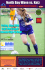### Origlio Beverage Satisfies Thirst for Productivity and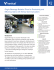### • Power and Intensity • Doppler effect for (i) mechanical waves e.g.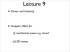### Aspen Airwaves - Aspen Flying Club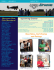### one sheet Dark Halo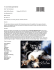### döfix No Sew, Inc. • 1947 N. Ironway Dr • Sanford, MI 48657 • Tel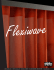### Two - Z-World LARP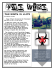### Wave loads on fixed offshore wind turbines